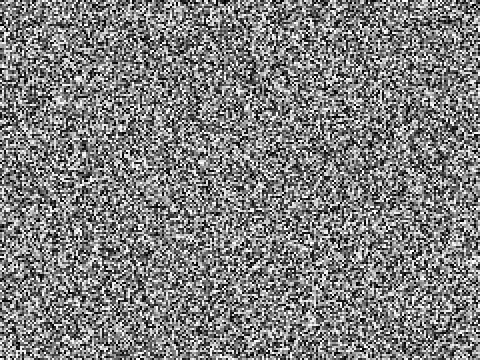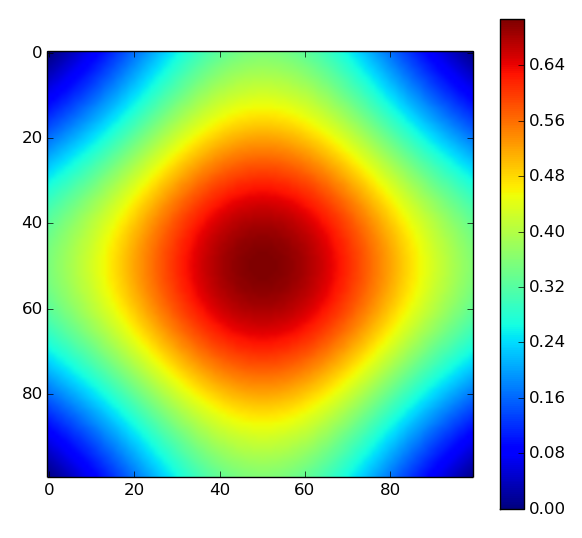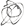Jun 20 2017

# The range of Perlin noise

TL;DR: The range is $$[-\sqrt{N/4}, \sqrt{N/4}]$$ where $$N$$ is the number of dimensions, assuming continuous unit gradient vectors.

## Intro

Lots of scenes in nature are random-looking: clouds, water, fire, grass. Sure, we can tell those apart, but each cloud is different from its neighbors.

Thus, in computer graphics, when we want to simulate nature, we need to generate some random numbers. However, if I just plotted a bunch of random numbers between 0 and 1, it would look like this:This is called white noise. Each pixel is independent from its neighbors. In graphics, we don’t want that, we want patterns that the eye can understand. We want coherent noise: a function that is random looking but locally sensitive. That is, if $$f(x, y) = c$$, then $$f(x + \epsilon, y + \epsilon) = c + \epsilon’$$. That is, a small move away from $$(x, y)$$ leads to a small change from $$c$$.

Such a function was developed by Ken Perlin in 1983 and he eventually got an academy award for it, because it was that useful to 3D graphics. In 2D, it looks like this:And it also comes in 1D and 3D versions. I won’t explain how Perlin noise is generated in this blog post, as there are already excellent resources for this. See link 1, link 2 and link 3. Rather, I want to address what might seem like a simple question: what is the range of values of Perlin noise?

## An under-addressed question

Why care about the range? Well, consider the simple task of plotting 2D Perlin noise in grayscale. You need to convert Perlin noise at every pixel into a value from 0 to 255, which is the input that the monitor takes. Ideally, the smallest and largest values of Perlin noise should map to 0 and 255 respectively. You could make an approximation and clamp values that fall outside that range, but it would be nice to get an exact range in advance.

Interestingly enough, this information is hard to find! None of the tutorials I found mention anything about the range. Not even the original paper does. As a result, there are a lot of misconceptions. The Unity implementation contradicts itself saying that the Mathf.PerlinNoise returns a “float Value between 0.0 and 1.0” yet warns that “it is possible for the return value to slightly exceed 1.0f”.

Now, I’m not the first person to ask this question. It’s been asked on Stack Overflow before and there’s a thread on GameDev.net from 2004 where the posters fumble around and eventually arrive at an answer (while making a bunch of wrong claims, such as saying that Perlin-noise is Gaussian-distributed and can have arbitrarily large values with low probability).

That’s why I’m hoping this blog post will provide a definite answer to this question. It might seem like a lot of work just to find a number but hey, I think that number is important.

## Intuition

It’s actually quite easy to guess the range of Perlin noise if you have a good visualization tool and knowledge of how Perlin noise is calculated. As a reminder, Perlin noise works by generating a random unit vector at each point of a lattice which represents the gradient of Perlin noise at that point (hence why it’s called gradient noise) and interpolating in-between.

If you use an interactive visualizer like the excellent one Andrew Kensler made and just play around arranging the vectors in different configurations, you can make spots become brighter or darker. And it looks like the configuration of vectors that achieve the brightest/darkest spot is when they all point towards/away from the center of a square.

This would make sense given that the Perlin noise equation involves a dot product between the gradient vectors at the corners and the delta vectors from the corners to the center. When all the gradient vectors point at the center, the dot product is maximized.

We can demonstrate that this configuration of vector indeed produces the largest Perlin noise value, using one of three different methods:

1. Randomly sampling points on Perlin noise by generating a random tuple. In the case of 2D Perlin noise, that would be (top-left angle, top-right angle, bottom-left angle, bottom-right angle, x, y)
2. Use numerical optimization (hill-climbing) to find the maximum spot on Perlin noise by treating it as a continuous function, which it is.
3. Algebraically find the maximum value of Perlin noise as a continuous function

## What kind of Perlin noise?

Before I proceed, I need to clarify what exactly I mean by Perlin noise, since it has a few variations and tweaks.

First, Perlin noise is often confused with value noise or fractal brownian motion. Determining the range of value noise is trivial and doesn’t require optimization techniques.

Second, Ken Perlin published an Improved Perlin noise where he made a few tweaks. He changed the original interpolation function from $$3t^2 - 2t^3$$ to $$6t^5 - 15t^4 + 10t^3$$ which has better continuity properties. This doesn’t affect the range of values of Perlin noise but it does affect the value and location of the maximum in the gradient magnitude.

Third, there are many different ways to select the random vectors at the grid cell corners. In Improved Perlin noise, instead of selecting any random vector, one of 12 vectors pointing to the edges of a cube are used instead. Here, I will talk strictly about a continuous range of angles since it is easier - however, the range of value of an implementation of Perlin noise using a restricted set of vectors will never be larger. Finally, the script in this repository assumes the vectors are of unit length. If they not, the range of value should be scaled according to the maximum vector length. Note that the vectors in Improved Perlin noise are not unit length.

Now, onto the meat of the work.

## Numerical optimization

I’m skipping random point sampling because numerical optimization is a better method when the function to optimize is differentiable (Perlin noise is differentiable). Random sampling is not an effective way to find the maximum value of a function, especially given that we have 6 different variables in 2D Perlin noise and 19 different variables in 3D Perlin noise to maximize over.

The key techniques used are gradient descent and automatic differentiation. Gradient descent (or ascent) just means “keep going up the hill until you’ve reached the top of the hill”. Perlin noise is made of many hills though, so hill-climbing is done multiple times, starting at random points, to have the best chance of finding the highest hill. However, hill-climbing works best if the “up” direction of the hill is known. This is determined using the gradient (derivative) of Perlin noise. This can be worked out analytically, but it’s cool to use automatic differentiation which calculates the derivative of any function with respect to its arguments. And I don’t mean function in the mathematical sense, I’m also talking about programming functions. You can differentiate a function even if it contains branches, loops and function calls!

You can find my implementation on Github. The end result for 2D Perlin noise does indeed tell me that the maximum is at the center of the square, with all gradient vectors pointing towards it.

Value 0.707107 at position (0.5, 0.5)
with angles [44.98, 134.99, 315.01, 225.0]
equivalently [0.25, 0.75, 1.75, 1.25]piSimilarly for 3D Perlin noise

Value 0.866025 at position (0.5, 0.5, 0.5)
with angles [44.98, 54.72, 134.99, 54.72, 315.01, 54.72, 225.0, 54.72]
equivalently [0.25, 0.304, 0.75, 0.304, 1.75, 0.304, 1.25, 0.304]pi


If we assume this result generalizes for n dimensions, then the maximum value of Perlin noise is indeed $$\sqrt{N/4}$$. Note that simulations would get expensive very quickly since n-dimensional Perlin noise has $$(n-1)2^n + n$$ variables to optimize over.

Note that we can use the same technique to find the maximum magnitude of the gradient of Perlin noise using this technique, by computing the gradient of the magnitude of the gradient, then hill-climbing. It’s very slow but it works.

## An algebraic derivation

Finally, we can finish off by showing that our intuition and the numerical result is correct using a formal proof.

Theorem

Suppose $$u(t)$$ is an easing function from $$[0, 1]$$ such that $$u(t)$$ is non-decreasing, $$u(t) \le t$$ on $$[0, \frac{1}{2}]$$ and $$u(t) \ge t$$ on $$[\frac{1}{2}, 1]$$.

Then the 2D Perlin Noise under an easing function $$u(t)$$ and unit Perlin vectors has values in the range of $$[-\sqrt{\frac{1}{2}}, \sqrt{\frac{1}{2}}]$$.

Proof

2D Perlin Noise function is defined on a 2D grid with random vector at each grid point representing the gradient of the function at that point. Within a grid cell, the function value is determined by the four unit vectors $$A, B, C, D$$ at its corners. It suffices to find the range of possible values within one cell to find the range of possible value within all cells.

Let

We can rewrite the dot product. Note that $$\norm{A} = \norm{B} = \norm{C} = \norm{D} = 1$$ since the Perlin vectors are unit vectors.

We want to find the maximum value of this equation over all possible corner vectors $$A, B, C, D$$ and positions $$x, y \in [0, 1]$$. Since Perlin Noise is symmetric, the minimum is obtained in the exact same way.

This function is dome-shaped, with a peak at the center. We want to prove that the maximum is at $$(\frac{1}{2},\frac{1}{2})$$. First, rearrange the terms to emphasize the order of interpolation

We start by maximizing $$f(y) = \norm{(x,y)} (1 - u(y)) + \norm{(x, y-1)} u(y)$$, independently of $$x$$. First, take the derivative.

Finding all the roots of $$f’(y)$$ on [0, 1] is difficult. However, we can show that $$f’(y) \ge 0$$ on $$[0, \frac{1}{2}]$$ and $$f’(y) \le 0$$ on $$[\frac{1}{2}, 1]$$.

First, we show that $$\norm{(x,y-1)} - \norm{(x,y)} \ge 0 \Longleftrightarrow y \le \frac{1}{2}$$.

Next, we show that $$\frac{y}{\norm{(x,y)}}(1 - u(y)) - \frac{1-y}{\norm{(x, y-1)}} u(y) \ge 0 \Longleftrightarrow y \le \frac{1}{2}$$.

Since $$u(y) \le y \Longleftrightarrow y \le \frac{1}{2}$$, then we just need to show

Which we already proved previously. Combinining these results, and the condition that $$u’(y) \ge 0$$, we get

for $$y \le \frac{1}{2}$$ and $$f’(y) \le 0$$ for $$y \ge \frac{1}{2}$$. This proves that $$y = \frac{1}{2}$$ maximizes $$f(y) = \norm{(x,y)} (1 - u(y)) + \norm{(x, y-1)} u(y)$$. With $$u(\frac{1}{2}) = \frac{1}{2}$$, we get

Which is maximized with $$x = \frac{1}{2}$$ using the same proof. The same principle can be repeated with higher-dimensional Perlin noise using induction by iteratively maximizing one dimension at a time.

The value of Perlin noise at $$(\frac{1}{2}, \frac{1}{2})$$ is $$(\frac{1}{2})(\frac{1}{2})\norm{(\frac{1}{2}, \frac{1}{2})} = \sqrt{\frac{1}{2}}$$. Note that Perlin noise is symmetric around 0 so the minimum value is $$-\sqrt{\frac{1}{2}}$$.

And this concludes our algebraic proof.

Corollary

The N-dimensional Perlin noise has values in the range of $$[-\sqrt{\frac{N}{4}}, \sqrt{\frac{N}{4}}]$$. It can be shown that the arrows must point to the center in N-dimension Perlin noise via induction reusing the steps of the 2D Perlin noise proof.